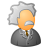# Generating a Spatial Weight Matrix using the TIME_SPACE concept

1238
0
01-15-2014 10:54 AMNew Contributor
Dear ESRI I have a question regarding the creation of a spatial weight matrix (SWM). I have a data set that contains 71 points depicting ozone values for two time periods (n = 142). When I create a SWM for each time period separately (i.e. independent of time) and run Moran???s I using the SWM as input I get the same results as my R Morans??? I. That is ArcGIS and R produce the same results in Morans??? I; hence, both methods must produce the same SWM. So this is a good check.

Now here is where things get interesting. When I create a SWM in ArcGIS using the concept of TIME_SPACE and run Morans???I I get different results when compared to my R program using Moran???s I. Since I have concluded that Moran???s I is calculated the same in both my R program and ArcGIS and all the parameters are set the same in ArcGIS and in R the issue must lies in the generation of the SWM under the concept TIME_SPACE. So my questions is how does ArcGIS combine the matrices generated from the 71 points over two time periods. My SWM generated in R is 142 rows by 142 columns. If I take the ArcGIS SWM convert it to a tables I get 11534 records. If my guess is true (e.g. every table record is a element in the matrix) then ArcGIS does not create a symmetrical matrix. That is the root of 11534 is 107.4

In simple terms how are the input matrix for the two time periods combine to create a single SWM under the TIME_SPACE concept.

Thanks
Mike
Tags (2)
0 Replies